# Improving High-Temperature Cycle Stability and Rate Performance of LiNi0.82Co0.11Mn0.07O2 Cathode Materials Using Hydrogen Peroxide Solution Washing System

Improving High-Temperature Cycle Stability and Rate Performance of LiNi0.82Co0.11Mn0.07O2 Cathode Materials Using Hydrogen Peroxide Solution Washing System

Department of Nano-Polymer Science & Engineering, Korea National University of Transportation, Chungju, Chungbuk 27469, Republic of Korea

Corresponding Author Email:
jt1234@ut.ac.kr
Page:
98-102
|
DOI:
https://doi.org/10.14447/jnmes.v25i2.a02
21 July 2021
|
Accepted:
5 May 2022
|
Published:
10 June 2022
| Citation

OPEN ACCESS

Abstract:

In this study, for the removal of residual lithium $\left(\mathrm{Li}_{2} \mathrm{CO}_{3}, \mathrm{LiOH}\right)$ from a nickel-rich cathode material surface, $LiNi_{0.82} \mathrm{Co}_{0.11} \mathrm{Mn}_{0.07 \mathrm{O}_{2}}$ cathode materials were washed with an aqueous solution of hydrogen peroxide $\left(\mathrm{H}_{2} \mathrm{O}_{2}\right) . \mathrm{H}_{2} \mathrm{O}_{2}$ ( $\left.\mathrm{pH} 6.04\right)$, a weak acid, can easily decompose $\mathrm{Li}_{2} \mathrm{CO}_{3}$ and $\mathrm{LiOH}$ as an oxidizing agent. On titration of residual lithium, the amounts of $\mathrm{LiOH}$ and $\mathrm{Li}_{2} \mathrm{CO}_{3}$ are 390 and $605 \mathrm{ppm}, 25$ - and 47 -times lower, after $\mathrm{H}_{2} \mathrm{O}_{2}$ washing compared to 10,296 and $28,440 \mathrm{ppm}$, respectively, in case of cathode materials before washing. On DSC thermal analysis, the peak temperature and calorific value of the cathode material washed with $\mathrm{H}_{2} \mathrm{O}_{2}$ were $245.5{ }^{\circ} \mathrm{C}$ and $602.0 \mathrm{~J} / \mathrm{g}$, respectively, whereas the bare case was $208.6{ }^{\circ} \mathrm{C}$ and $1,071 \mathrm{~J} / \mathrm{g}$, respectively. Therefore, $\mathrm{H}_{2} \mathrm{O}_{2}$-washed $\mathrm{LiNi}_{0.82} \mathrm{Co}_{0.11} \mathrm{Mn}_{0.07} \mathrm{O}_{2}$ cathode materials had higher capacity heat retention after 100 cycles at $55{ }^{\circ} \mathrm{C}(85.6 \%$ at $0.5 \mathrm{C})$ than the bare $\mathrm{LiNi}_{0.82} \mathrm{Co}_{0.11} \mathrm{Mn}_{0.07} \mathrm{O}_{2}$ cathode materials $(78.6 \%$ at $0.5 \mathrm{C})$.

Keywords:

Ni-rich materials, Li residuals, H2O2, washing process

1. Introduction

The Lithium-ion batteries (LIBs) have gained increased research attention as the most advanced energy storage devices being widely used in portable electronics and electric vehicles. [1,2] These applications require further energy density promotion and cost reduction in LIBs, which are mainly determined by the cathode materials.  Therefore, materials with Ni contents greater than $80 \%$ are the most favorable cathode materials for EV applications owing to their high capacity $(\sim 220 \mathrm{mAh} / \mathrm{g})$ and low manufacturing costs compared to conventional $\mathrm{LiCoO}_{2}$ materials. [4-6]

However, major drawbacks of structural degradation, gas evolution, cation mixing, and the presence of a large amount of $\mathrm{Li}_{2} \mathrm{CO}_{3}$ or $\mathrm{LiOH}$ on the surface must be overcome for successful commercialization. [7-11] The removal of $\mathrm{Li}$ residuals from Ni-rich materials is a key problem that needs to be solved because the presence of $\mathrm{Li}$ residuals poses the potential risk of gas evolution on the surface. [12,13]

To address these problems in the industrial field, a waterwashing process is introduced to reduce Li residues. However, Tasaki et al.  reported that $\mathrm{Li}_{2} \mathrm{CO}_{3}$ in water ( $1.3 \mathrm{~g}$ per 100 $\mathrm{mL})$ has lower solubility than $\mathrm{LiOH}(12.8 \mathrm{~g}$ per $100 \mathrm{~mL})$ at $25{ }^{\circ} \mathrm{C}$, although it possesses excellent electrochemical inertness, the exposed $\mathrm{Li}_{2} \mathrm{CO}_{3}$ particles easily react with $\mathrm{LiPF}_{6}$ based electrolyte and $\mathrm{LiPF}_{6}$ powder to generate $\mathrm{LiF}, \mathrm{CO}_{2}$, and $\mathrm{POF}_{3}$.  Therefore, it is important to simultaneously remove $\mathrm{LiOH}$ and $\mathrm{Li}_{2} \mathrm{CO}_{3}$ to enable the commercialization of nickel-rich cathode materials. Park et al.  succeeded in reducing the amount of $\mathrm{Li}_{2} \mathrm{CO}_{3}$ to $2,890 \mathrm{ppm}$ and $\mathrm{LiOH}$ to 5,800 ppm by using a nitric acid aqueous solution solvent evaporation process. Park et al.  selected a water/active substance (1:1 ratio) and a drying temperature of $120^{\circ} \mathrm{C}$ as the optimal washing condition to reduce the total residual lithium content by $1 / 3$ while minimizing cell performance degradation. However, until now, although research results for removing residual lithium have been effective at $25^{\circ} \mathrm{C}$, concerns about thermal stability at high temperatures $\left(>50{ }^{\circ} \mathrm{C}\right)$ remain.

To solve this problem, in this study, $\mathrm{LiNi} 0.82 \mathrm{Co}_{0.11} \mathrm{Mn}_{0.07} \mathrm{O}_{2}$ cathode materials were washed with an aqueous solution of $\mathrm{H}_{2} \mathrm{O}_{2} \cdot \mathrm{H}_{2} \mathrm{O}_{2}$ (pH 6.04), a weak acid, can easily decompose $\mathrm{Li}_{2} \mathrm{CO}_{3}$ and $\mathrm{LiOH}$ as an oxidizing agent. It effectively removes $\mathrm{LiOH}$ and reacts with $\mathrm{Li}_{2} \mathrm{CO}_{3}$, given by reactions (1) and (2)  for removal of residual lithium.

To solve this problem, in this study, $\mathrm{LiNi} 0 .{ }_{82} \mathrm{Co}_{0.11} \mathrm{Mn}_{0.07} \mathrm{O}_{2}$ cathode materials were washed with an aqueous solution of $\mathrm{H}_{2} \mathrm{O}_{2} \cdot \mathrm{H}_{2} \mathrm{O}_{2}$ (pH 6.04), a weak acid, can easily decompose $\mathrm{Li}_{2} \mathrm{CO}_{3}$ and $\mathrm{LiOH}$ as an oxidizing agent. It effectively removes $\mathrm{LiOH}$ and reacts with $\mathrm{Li}_{2} \mathrm{CO}_{3}$, given by reactions (1) and (2)  for removal of residual lithium.

$\mathrm{Li}_{2} \mathrm{CO}_{3}+\mathrm{H}_{2} \mathrm{O}_{2} \rightarrow 2 \mathrm{LiOH}+\mathrm{CO}_{2}+1 / 2 \mathrm{O}_{2}$      (1)

$\mathrm{LiOH}(\mathrm{aq}) \rightarrow \mathrm{H}+(\mathrm{aq})+\mathrm{OH}-(\mathrm{aq})$      (2)

To the best of our knowledge, no study has reported a $\mathrm{H}_{2} \mathrm{O}_{2}$ aqueous solution washing process for the removal of residual lithium from $\mathrm{LiNi}{ }_{0.82} \mathrm{Co}_{0.11} \mathrm{Mn}_{0.07} \mathrm{O}_{2}$ cathode materials.

2. Experiment

2.1 Preparation of $\mathrm{LiNi}{ }_{0.82} \mathrm{Co}_{0.11} \mathrm{Mn}_{0.07} \mathrm{O}_{2}$

A commercially available $\mathrm{LiNi}_{0.82} \mathrm{Co}_{0.11} \mathrm{Mn}_{0.07}(\mathrm{OH})_{2}$ precursor (ECOPRO Corp., Korea) was ground with a stoichiometric amount of $\mathrm{LiOH} \cdot \mathrm{H}_{2} \mathrm{O}$. Subsequently, the pristine NCM material was obtained after the ground material was calcined at $750{ }^{\circ} \mathrm{C}$ for $15 \mathrm{~h}$ in $\mathrm{O}_{2}$.

2.2 Washing process of $\mathrm{LiNi}{ }_{0.82} \mathrm{Co}_{0.11} \mathrm{Mn}_{0.07} \mathrm{O}_{2}$

For the $\mathrm{H}_{2} \mathrm{O}_{2}$ washing process, $1 \mathrm{~g}$ of $\mathrm{LiNi} 0_{0.82} \mathrm{Co}_{0.11} \mathrm{Mn}_{0.07} \mathrm{O}_{2}$ powder (NCM) was dissolved in $100 \mathrm{~g}$ of solvents, one of which was DI water and the other was a mixture of DI water and $\mathrm{H}_{2} \mathrm{O}_{2}(0.01 \mathrm{M})$. The solution of $\mathrm{NCM}$ powder and solvent was stirred for $60 \mathrm{~min}$ and dried at $120^{\circ} \mathrm{C}$ until the solvent completely evaporated. $\mathrm{H}_{2} \mathrm{O}_{2}$-washed $\mathrm{NCM}$ and water-washed $\mathrm{NCM}$ were prepared after the solvent-dried NCM powders were heated at $750^{\circ} \mathrm{C}$ for $10 \mathrm{~h}$ under $\mathrm{O}_{2}$ flow.

2.3 Material characterization

X-ray diffraction patterns for the cathodes were obtained using a Siemens D-5000 diffractometer in the $2 \theta$ range of $10^{\circ}$ to $80^{\circ}$ with $\mathrm{Cu} \mathrm{K} \alpha$ radiation $(\lambda=1.5406 \overset{\circ }{\mathop{\text{A}}}\,)$. The obtained powder morphology was observed using scanning electron microscopy (SEM, JSM-7610F, JEOL). The surface area and surface morphology of the obtained powders were observed using an atomic force microscope (N3-AM, Bruker).

2.4 Electrochemical characterization

To prepare the positive electrode, $0.8 \mathrm{~g}$ $\mathrm{LiNi}_{0.82} \mathrm{Co}_{0.11} \mathrm{Mn}_{0.07} \mathrm{O}_{2}$ powder $0.1 \mathrm{~g}$ Super-P (Aldrich), Nmethyl-2-pyrrolidone (NMP), and $0.1 \mathrm{~g}$ PVDF (Kureha KF100) binder were mixed. Subsequently, the slurry was applied on aluminum foil using a doctor blade to form a film (thickness, $25 \mu \mathrm{m}$ ). The electrode was dried at $120^{\circ} \mathrm{C}$ for $4 \mathrm{~h}$ in a vacuum-drying oven. The CR2032-type coin cell was combined in a glovebox using a $\mathrm{LiNi}_{0.82} \mathrm{Co}_{0.11} \mathrm{Mn}_{0.07} \mathrm{O}_{2}$ cathode electrode, lithium metal (as anode), porous polypropylene, and a $1 \mathrm{M}$ $\mathrm{LiPF} 6$ solution with a $7: 3$ volume ratio of dimethyl carbonate $(\mathrm{DMC})$ /ethylene carbonate (EC). The electrodes were charged and discharged galvano-statically from $3.0$ to $4.3 \mathrm{~V}$ versus $\mathrm{Li}^{2} / \mathrm{Li}^{+}$at a continuous current of 85 $\mathrm{mAg}^{-1}(0.5 \mathrm{C})$. Cycle performances at $55^{\circ} \mathrm{C}$ were evaluated at $85 \mathrm{mAg}^{-1}(0.5 \mathrm{C})$.

3. Results and Discussion

Figure 1 shows SEM images of the bare and $\mathrm{H} 2 \mathrm{O} 2$-washed cathode materials. Notably, the particles adopt a spherical morphology in the secondary particles and the estimated particle diameter is approximately $13-15 \mu \mathrm{m}$, as shown in Figure 1 (a, d). Figure 1 (b, e)$shows that the primary particle shapes were not clearly identified. Figure 1 (c) shows that the primary particles are rounded with many large protrusions on the surface; however, Figure 1 (f) shows they are right-angled with fine-sized protrusions. ## 1.png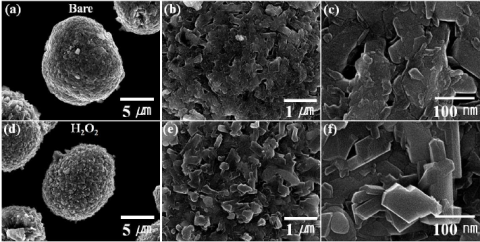Figure 1. SEM images of the$(\mathrm{a}-\mathrm{c})$bare and$(\mathrm{d}-\mathrm{f}) \mathrm{H}_{2} \mathrm{O}_{2}$washed cathode materials Figure 2 (a, b) shows AFM images of the bare and$\mathrm{H}_{2} \mathrm{O}_{2}$-washed cathode materials. Compared with the bare sample, the average roughness$\left(\mathrm{R}_{\mathrm{a}}\right)$after$\mathrm{H}_{2} \mathrm{O}_{2}$-washing increased from 36 to$83 \mathrm{~nm}$. This increased surface area is owing to the removal of residual lithium from the cathode materials surface. ## 2.png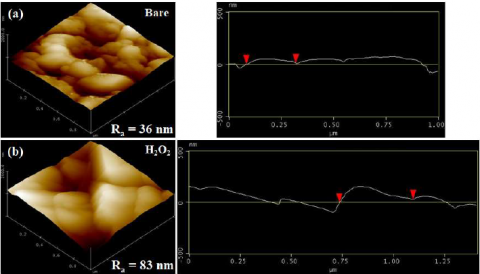Figure 2. AFM images of the (a) bare and (b)$\mathrm{H}_{2} \mathrm{O}_{2}$-washed cathode materials Figure 3 shows XRD patterns of the bare and$\mathrm{H}_{2} \mathrm{O}_{2}$-washed cathode materials. All peaks correspond to the layered$\alpha\mathrm{NaFeO}_{2}$structure of the space group$\mathrm{R}-3 \mathrm{~m}$without any impurities. The$\mathrm{H}_{2} \mathrm{O}_{2}$-washed cathode had a larger c-axis lattice parameter than the bare clathode. The lithium in the structure is presumed to be desorbed during the$\mathrm{H}_{2} \mathrm{O}_{2}$washing process, thereby increasing the repulsive force between oxygen in the cathode structure. Owing to the reduction of lithium in the structure, the cation-mixing phenomenon in the structure decreased and the peak value of$\mathrm{I}_{(003)} / \mathrm{I}_{(104)}$increased after$\mathrm{H}_{2} \mathrm{O}_{2}$washing. However, after the$\mathrm{R}$-factor analysis, which determines the degree of crystallinity of hexagonal, the structure became well-ordered after washing with$\mathrm{H}_{2} \mathrm{O}_{2}$was confirmed. ## 3.png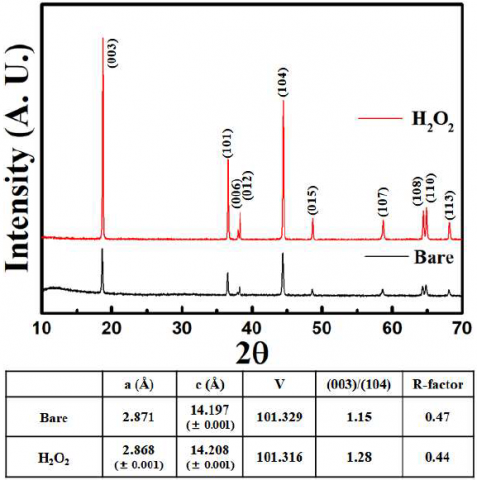Figure 3. XRD analysis of the bare and$\mathrm{H}_{2} \mathrm{O}_{2}$-washed cathode materials The Li residuals amount is closely related to the cationmixing behavior because the unoccupied Li ions in the Li layer can be transformed into Li residuals. The amounts of$\mathrm{LiOH}$and$\mathrm{Li}_{2} \mathrm{CO}_{3}$are calculated and obtained using equations (3) and (4) [19,20]:$\mathrm{LiOH}+\mathrm{HCl} \rightarrow \mathrm{LiCl}+\mathrm{H}_{2} \mathrm{O}$(3)$\mathrm{Li}_{2} \mathrm{CO}_{3}+2 \mathrm{HCl} \rightarrow 2 \mathrm{LiCl}+\mathrm{H}_{2} \mathrm{O}+\mathrm{CO}_{2}$(4) Li residuals amounts in the pristine and coated samples are listed in Table 1. The LiOH amount is$390 \mathrm{ppm}$, which is 25 times lower after$\mathrm{H}_{2} \mathrm{O}_{2}$washing compared to 10,296 ppm of the cathode materials before washing, and$\mathrm{Li}_{2} \mathrm{CO}_{3}$is$605 \mathrm{ppm}$, which is 47-times lower after washing compared to 28,440 ppm of the cathode materials before washing. It is noted that the total amount of Li residuals in the reheated NCMs are subjected to the$\mathrm{H}_{2} \mathrm{O}_{2}$washing process. The amounts of$\mathrm{LiOH} / \mathrm{Li}_{2} \mathrm{CO}_{3}$used to indicate residual lithium and oxygen from the bare and$\mathrm{H}_{2} \mathrm{O}_{2}$-washed$\mathrm{NCM}$lattices (O lattice) that were present throughout the active material were investigated by X-ray photoelectron spectroscopy (XPS) profiles; the results are shown in Figure 4(a). The lower binding energy peak of$528.8 \mathrm{eV}$was assigned to the$\mathrm{O}$lattice and higher binding energy peak of$531 \mathrm{eV}$was assigned to residual lithium species, such as$\mathrm{Li}_{2} \mathrm{CO}_{3}$and$\mathrm{LiOH}$. After$\mathrm{H}_{2} \mathrm{O}_{2}$washing, the peak amount around$531 \mathrm{eV}$decreased owing to the reduced residual lithium. Additionally, it was confirmed that the peak amount indicating the amount of$\mathrm{O}$lattice around$528.8 \mathrm{eV}$increased as the surface residual lithium layer thickness decreased. The$\mathrm{C}$1s spectra collected on the high-Ni materials contain species from$\mathrm{Li}_{2} \mathrm{CO}_{3}(289.8\mathrm{eV}$) and adventitious carbon (centered near$284.8 \mathrm{eV}$and$286.0 \mathrm{eV})$, as shown in Figure 4(b).  ## 4.png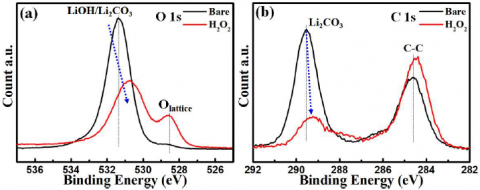Figure 4. XPS spectra of (a) O 1s and (b) C 1s of the bare and$\mathrm{H}_{2} \mathrm{O}_{2}$-washed cathode materials The charge-discharge curves of the bare and$\mathrm{H}_{2} \mathrm{O}_{2}$-washed cathode materials were evaluated at a current density of 17$\mathrm{mA} / \mathrm{g}$at$55^{\circ} \mathrm{C}$in the voltage range of$3.0-4.3 \mathrm{~V}$, as shown in Figure 5(a). The discharge capacity of bare and$\mathrm{H}_{2} \mathrm{O}_{2}$-washed cathode materials is$209.7$and$214.8 \mathrm{mAh} / \mathrm{g}$, respectively. The discharge capacity of the$\mathrm{H}_{2} \mathrm{O}_{2}$-washed material was higher than that of the bare material. The increased discharge capacity was due to the increased surface area after$\mathrm{H}_{2} \mathrm{O}_{2}$washing. To confirm reduced lithium residual effect during cycling, the$\mathrm{dQ} / \mathrm{dV}$profiles of both the cathode materials were obtained by differentiating the charge-discharge curves, as shown in Figure 5(b). As the impediment to lithium-ion migration was reduced owing to the removal of the surface residual lithium, the overvoltage of the initial oxidation peak during charging decreased after$\mathrm{H}_{2} \mathrm{O}_{2}$washing. Therefore, the discharge capacity has increased and the rate performances are expected to improve. ## 5.png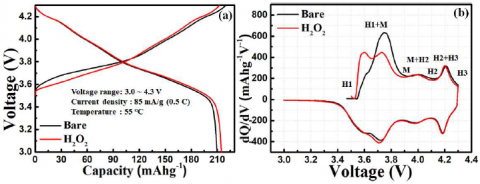Figure 5. (a) Initial charge-discharge and (b) differential capacity$(d \mathrm{Q} / \mathrm{dV})$curves of bare and$\mathrm{H}_{2} \mathrm{O}_{2}$-washed cathode materials Figure 6(a) shows the cycling performance of the bare and$\mathrm{H}_{2} \mathrm{O}_{2}$-washed cathode materials at$55^{\circ} \mathrm{C}$. Although the cell based on Bare retained only$78.6 \%$of its initial capacity after 100 cycles, the$\mathrm{H}_{2} \mathrm{O}_{2}$-washed cathode materials showed a capacity retention of$85.6 \%$. This result suggests that the side reaction between the residual lithium and the electrolyte after washing with$\mathrm{H}_{2} \mathrm{O}_{2}$was suppressed. Rate capability is an important electrochemical characteristic of Li-ion batteries for electric and hybrid electric vehicles. Figure 6(b) shows the rate capability of the bare and$\mathrm{H}_{2} \mathrm{O}_{2}$-washed cathode materials at$55^{\circ} \mathrm{C}$. The$\mathrm{H}_{2} \mathrm{O}_{2}$-washed cathode materials exhibited higher capacity than the bare and Al-doped electrodes$(0.5 \mathrm{C})$, which further demonstrates the enhanced rate performance. The discharge capacity of the bare is presumed high before$0.5 \mathrm{C}$because the content of lithium is higher than the result of$\mathrm{H}_{2} \mathrm{O}_{2}$washing. After$0.5 \mathrm{C}$, the rate performances were enhanced owing to the reduced residual lithium effect after washing with$\mathrm{H}_{2} \mathrm{O}_{2}$. The side reaction products have more negative effect of the cell performances. The benefit effect of the washing at high rate than at low rate. After 35 cycles, the cells maintained$93.7 \%$its initial capacity (without washing) and$97.9 \%$its initial capacity (after washing) at$0.1 \mathrm{C}$. The improvement in rate stability could explained by the washing process which removed residual lithium in cathode material. ## 6.png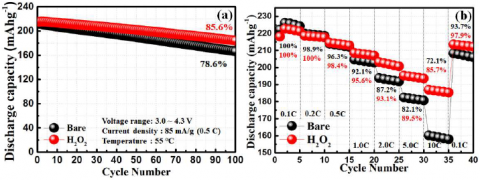Figure 6. (a) Cycle performance and (b) rate capability of bare and$\mathrm{H}_{2} \mathrm{O}_{2}$-washed cathode materials Figure 7(a) and (b) show the$A C$impedance spectrum and the difference in charge transfer resistance as a function of voltage of bare and$\mathrm{H}_{2} \mathrm{O}_{2}$-washed cathode materials. The electrode data show$R_{S E I}$and$R_{c t}$, indicating solid electrolyte interphase resistance ($R_{\text {SEI }}$) and charge transfer resistance$\left(R_{c t}\right)$. The semicircle at low frequencies reflects the$R_{c t}$and interfacial capacitance between the electrodes and electrolyte, and the semicircle at high frequencies reflects the charge transfer resistance.${ }^{21,22}$We can obtain information on the cathode surface during cycling from the second semicircle, which reflects$R_{\text {ct. }}$It is clear from Figure 7(b) that a pronounced difference appears at the$\mathrm{R}_{\mathrm{ct}}$of$4.3 \mathrm{~V} . \mathrm{H}_{2} \mathrm{O}_{2}$-washed cathode materials exhibit low$R_{c t}$in all voltage ranges compared to bare cathode materials, as shown in Figure 7 . ## 7.png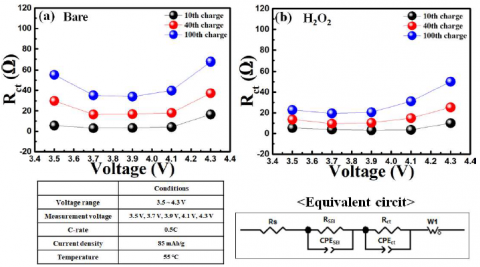Figure 7. Difference in charge transfer resistance as a function of voltage of (a) bare and (b)$\mathrm{H}_{2} \mathrm{O}_{2}$-washed cathode electrodes Figure 8 shows the DSC profiles of the bare and$\mathrm{H}_{2} \mathrm{O}_{2}$-washed cathode materials charged to$4.3 \mathrm{~V}$in the presence of a 1 M$\mathrm{LiPF}_{6} / \mathrm{EC}: \mathrm{DEC}$electrolyte. For the bare material, the onset temperature of the exothermic reaction peak was$208.6{ }^{\circ} \mathrm{C}$. In contrast, the$\mathrm{H}_{2} \mathrm{O}_{2}$-washed cathode materials showed higher onset temperatures and reduced heat generation compared to the bare cathode material. The high thermal stability of the$\mathrm{H}_{2} \mathrm{O}_{2}$-washed cathode materials suggests that the reduced residual lithium effectively suppresses the exothermic reaction. ## 8.pngFigure 8. DSC traces of the bare and$\mathrm{H}_{2} \mathrm{O}_{2}$-washed cathode materials charged to 4.3V 4. Conclusions$\mathrm{LiNi}_{0.82} \mathrm{Co}_{0.11} \mathrm{Mn}_{0.07} \mathrm{O}_{2}$cathode materials were washed with a$\mathrm{H}_{2} \mathrm{O}_{2}$aqueous solution. The residual amount of$\mathrm{LiOH}$and$\mathrm{Li}_{2} \mathrm{CO}_{3}$were 390 and$605 \mathrm{ppm}$, which is 25 - and 47 -times lower after$\mathrm{H}_{2} \mathrm{O}_{2}$washing, compared to 10,296 and$28440 \mathrm{ppm}$, respectively, in the cathode materials before washing.$\mathrm{R}_{\mathrm{ct}}$decreased in all voltage ranges during charging owing to the reduced residual lithium after$\mathrm{H}_{2} \mathrm{O}_{2}$washing. The discharge capacity of bare and$\mathrm{H}_{2} \mathrm{O}_{2}$-washed cathode materials was$209.7$and$214.8 \mathrm{mAh} / \mathrm{g}$, respectively. The$\mathrm{H}_{2} \mathrm{O}_{2}$-washed$\mathrm{LiNi}_{0.82} \mathrm{Co}_{0.11} \mathrm{Mn}_{0.07} \mathrm{O}_{2}$cathode materials exhibited higher capacity retention after 100 cycles at$55^{\circ} \mathrm{C}(85.6 \%$at$0.5 \mathrm{C})$than the bare$\mathrm{LiNi}_{0.82} \mathrm{Co}_{0.11} \mathrm{Mn}_{0.07 \mathrm{O}_{2}}$cathode materials$(72 \%$at$0.5 \mathrm{C})$. DSC thermal analysis results showed that the peak temperature and calorific value of the cathode material washed with$\mathrm{H}_{2} \mathrm{O}_{2}$were$245.5^{\circ} \mathrm{C}$and$602.0 \mathrm{~J} / \mathrm{g}$, respectively, whereas in case of the bare material, these were$208.6^{\circ} \mathrm{C}$and$1071 \mathrm{~J} / \mathrm{g}$, respectively. The high thermal stability of the$\mathrm{H}_{2} \mathrm{O}_{2}$-washed cathode materials suggests that the reduced residual lithium effectively suppresses exothermic reactions. Acknowledgment This study was supported by a grant from the Ministry of SMEs and Startups, Republic of Korea (S3045542) and the National Research Foundation of Korea (NRF) funded by the Korean Government (MSIT, No. This study was supported by the Ministry of SMEs and Startups, Republic of Korea (S3045542); the Technology Innovation Program (20003747, Development of high-performance cathode material manufacturing technology through valuable metal upcycling from waste batteries and waste cathode material) funded by the Ministry of Trade, Industry & Energy (MOTIE, Korea); and the Korea Agency for Infrastructure Technology; the National Research Foundation of Korea(NRF) funded by the Korea government (MSIT) (No.2021R1F1A1063481) and Korea Institute for Advancement of Technology(KIAT) grant funded by the Korea Government(MOTIE)(P0020614, HRD Program for Industrial Innovation). Nomenclature ${ }^{\circ} \mathrm{C}$temperature$\mathrm{g}$weight$\mathrm{V}$Voltage Greek symbols$\lambda$wavelength$\theta$angle$\mu\$ Micro- (one millionth)
References

 W. Liu, P. Oh, X. Liu, M. J. Lee, W. R. Cho, S. J. Chae, Y. S. Kim and J. Cho, Angew Chem Int Ed., 54 (15), 4440-4457 (2015)

 Y. Xia, J. Zheng, C. Wang and M. Gu, Nano Energy., 49, 434-452 (2018)

 W. Zhao, L. Zou, J. Zheng, H. Jia, J. Song, M. H. Engelhard, C. Wang, W. Xu, Y. Yang and J. Zhang, Adv Energy Mater., 11 (13), 2211-2220 (2018)

 X. Li, K. Zhang, D. Mitlin, Z. Yang, M. Wang, Y. Tang, F. Jiang, Y. Du and J. Zheng, Chem Mater. 30 (8), 2566-2573 (2018)

 D. W. Wang, R. H. Kou, Y. Yang, C. J. Sun, H. Zhao, M.J. Zhang, Y. Li, H.A. Huq, J. Y. P. Ko, F. Pan, Y. K. Sun, Y. Yang, K. Amine, J. Bai, Z. H. Chen and F. Wang, Adv Mater. 29, 160675 (2017).

 J. B. Goodenough and Y. S. Kim, Chem Mater. 22 (3), 587-603 (2010)

 C. Liu, F. Li, LP. Ma and H. M. Cheng, Adv Mater. 22 (8), E28-E62 (2010)

 N. Yabuuchi and T. Ohzuku, J Power Sources. 119 (21), 171-174 (2003)

 C. Li, H.P. Zhang, L. J. Fu, H. Liu, Y.P. Wu, E. Rahm, R. Holze and H.Q. Wu, Electrochim Acta. 51 (19), 3872-3883 (2006)

 Y. Kim, H. S. Kim and S. W. Martin, Electrochim Acta. 52 (3), 1316-1322 (2006)

 N. Yabuuchi, K. Yoshii, S. T. Myung, I. Nakai and S. Komaba, J Am Chem Soc. 133 (12), 4404-4419 (2011)

 J. H. Jo, C. H. Jo, H. Yashiro, S. J. Kim and S. T. Myung, J Power Sources. 313, 1-8 (2016)

 X. Xiong, Z. Wang, P. Yue, H. Guo, F. Wu, J. Wang and X. Li, J Power Sources. 222, 318-325 (2013)

 K. Tasaki, A. Goldberg, J. J. Lian, M. Walker, A. Timmons and S. J. Harris, J Electrochem Soc. 156 (12), 1019 (2009)

 Y. Bi, T. Wang, M. Liu, R. Du, W. Yang, Z. Liu, Z. Peng, Y. Liu, D. Wang and X. Sun,  RSC Adv. 6 (23), 19233-19237 (2016)

 J. H. Park, B. J. Choi, Y. S. Kang, S. Y. Park, D. J. Yun, I.S. Park, J. H. Shim, J. H. Park, H. N. Han and K. J. Park, Energy Technol. 6 (7), 1361-1369 (2018)

 K. Park, J. H. Park, S. G. Hong, B. Choi, S. Heo, S. W. Seo, K. M. Min and J. H. Park, Sci Rep. 7 (1), 44557 (2017)

 J. K. Yang, Y. H. Jin, D. H. Yang and D. W. Kim, J Korean Cryst Growth Cryst Technol. 29 (5), 222-228 (2019)

 J. Ge, L. Zhang, J. Lu, J. Zhu and S. Jiao, J Electrochem Soc. 163 (10), 300-304 (2016)

 Swonger L. Producing lithium. U.S Patent Application US2015/0014184A. Vol. 1; 2015.

 L. Cai, Z. Liu, K. An and C. Liang, J Electrochem Soc. 159 (7), 924-928 (2012)

 R. Stoyanova, E. Zhecheva, E. Kuzmanova, R. Alcántara, P. Lavela and J. L. Tirado, Solid State Ionics. 128 (1-4), 1-10 (2000)